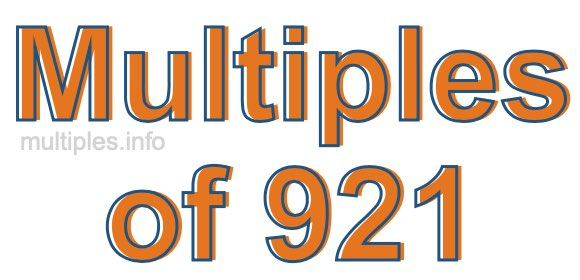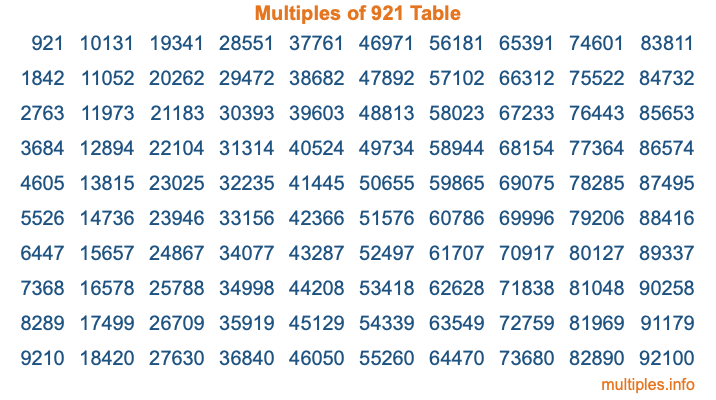Multiples of 921Welcome to the Multiples of 921 page. Here we will first teach you everything you will ever need to know about the multiples of 921, and then give you a study guide summary of everything we taught you to make sure you remember it all. Use this page to look up facts and learn information about the multiples of 921. This page will make you a multiples of nine hundred twenty-one expert!

Definition of Multiples of 921
Multiples of 921 are all the numbers that when divided by 921 equal an integer. Each of the multiples of 921 are called a multiple. A multiple of 921 is created by multiplying 921 by an integer.

Therefore, to create a list of multiples of 921, you start with 1 multiplied by 921, then 2 multiplied by 921, then 3 multiplied by 921, and so on for as long as you want. Thus, the list of the first five multiples of 921 is 921, 1842, 2763, 3684, and 4605. To see a larger list of multiples of 921, see the printable image of Multiples of 921 further down on this page. We also have a category where you can choose any nth multiple of 921.

Multiples of 921 Checker
The Multiples of 921 Checker below checks to see if any number of your choice is a multiple of 921. In other words, it checks to see if there is any number (integer) that when multiplied by 921 will equal your number. To do that, we divide your number by 921. If the the quotient is an integer, then your number is a multiple of 921.

Is  a multiple of 921?

Least Common Multiple of 921 and ...
A Least Common Multiple (LCM) is the lowest multiple that two or more numbers have in common. This is also called the smallest common multiple or lowest common multiple and is useful to know when you are adding our subtracting fractions. Enter one or more numbers below (921 is already entered) to find the LCM.

Check out our LCM Calculator if you need more details about the Least Common Multiple or if you need the LCM for different numbers for adding and subtraction fractions.

nth Multiple of 921
As we stated above, 921 is the first multiple of 921, 1842 is the second multiple of 921, 2763 is the third multiple of 921, and so on. Enter a number below to find the nth multiple of 921.

th multiple of 921

Multiples of 921 vs Factors of 921
921 is a multiple of 921 and a factor of 921, but that is where the similarities end. All postive multiples of 921 are 921 or greater than 921. All positive factors of 921 are 921 or less than 921.

Below is the beginning list of multiples of 921 and the factors of 921 so you can compare:

Multiples of 921: 921, 1842, 2763, 3684, 4605, etc.

Factors of 921: 1, 3, 307, 921

As you can see, the multiples of 921 are all the numbers that you can divide by 921 to get a whole number. The factors of 921, on the other hand, are all the whole numbers that you can multiply by another whole number to get 921.

It's also interesting to note that if a number (x) is a factor of 921, then 921 will also be a multiple of that number (x).

Multiples of 921 vs Divisors of 921
The divisors of 921 are all the integers that 921 can be divided by evenly. Below is a list of the divisors of 921.

Divisors of 921: 1, 3, 307, 921

The interesting thing to note here is that if you take any multiple of 921 and divide it by a divisor of 921, you will see that the quotient is an integer.

Multiples of 921 Table
Below is an image of the first 100 multiples of 921 in a table. The table is in chronological order, column by column. The first column has the first ten multiples of 921, the second column has the next ten multiples of 921, and so on.The Multiples of 921 Table is also referred to as the 921 Times Table or Times Table of 921. You are welcome to print out our table for your studies.

Negative Multiples of 921
Although not often discussed or needed in math, it is worth mentioning that you can make a list of negative multiples of 921 by multiplying 921 by -1, then by -2, then by -3, and so on, to get the following list of negative multiples of 921:

-921, -1842, -2763, -3684, -4605, etc.

Multiples of 921 Summary
Below is a summary of important Multiples of 921 facts that we have discussed on this page. To retain the knowledge on this page, we recommend that you read through the summary and explain to yourself or a study partner why they hold true.

There are an infinite number of multiples of 921.

A multiple of 921 divided by 921 will equal a whole number.

921 divided by a factor of 921 equals a divisor of 921.

The nth multiple of 921 is n times 921.

The largest factor of 921 is equal to the first positive multiple of 921.

921 is a multiple of every factor of 921.

921 is a multiple of 921.

A multiple of 921 divided by a divisor of 921 equals an integer.

921 divided by a divisor of 921 equals a factor of 921.

Any integer times 921 will equal a multiple of 921.

Multiples of a Number
Here you can get the multiples of another number, all with the same attention to detail as we did for multiples of 921 on this page.

Multiples of
Multiples of 922
Did you find our page about multiples of nine hundred twenty-one educational? Do you want more knowledge? Check out the multiples of the next number on our list!